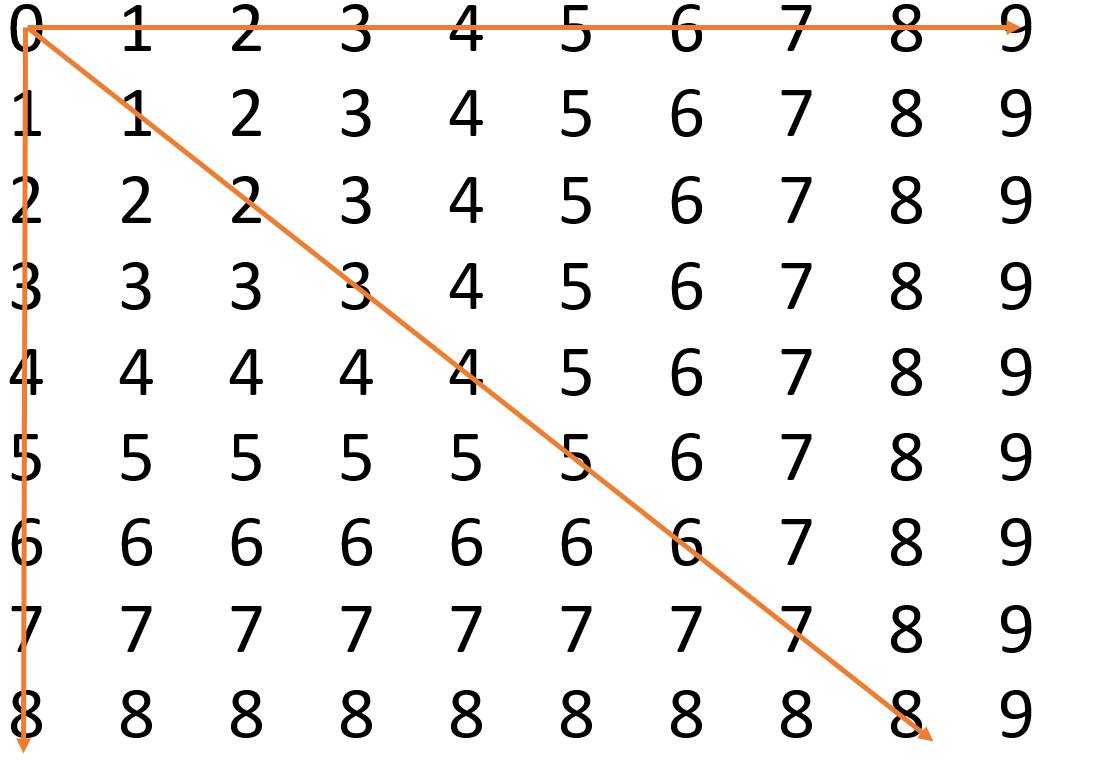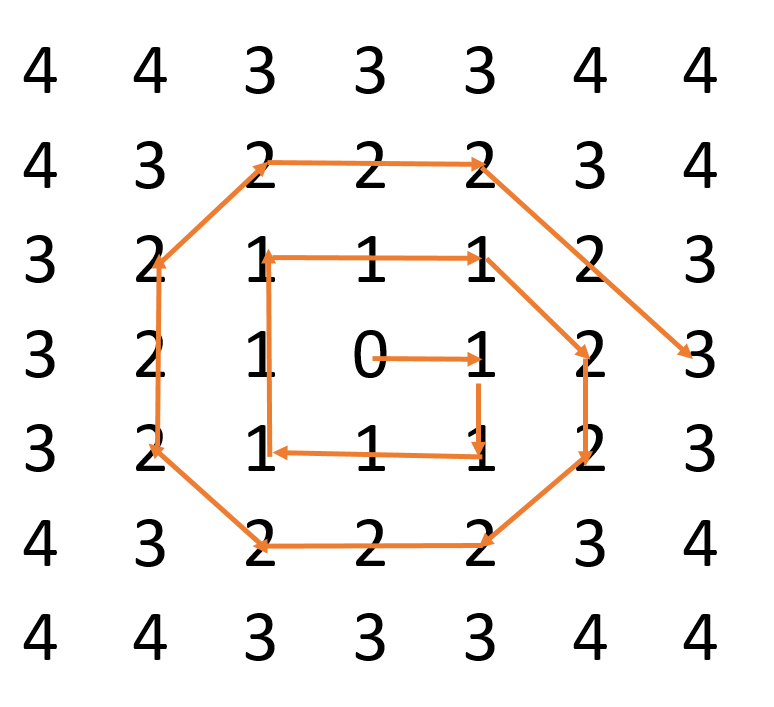# How can I make a sphere to all directions starting from a central point?

This sounds like an elementary school problem but I have a problem that I can’t solve. I want to find out how you can make a structure that expands evenly to all directions (like with the big bang) from a central fixed point. Knowing this would answer questions like; how did the universe expand from one point to a “spherical” filled structure? Here is a metaphor that exemplifies the problem: “Suppose you stand at the origin of the axes (x,y,z) with a hosepipe turned on. How do you fill the area around you to the shape of a (perfect) sphere?” I’m not a mathematician, what might contribute to the fact that I can’t solve it. Can you help me?

Below, I will describe what I tried through some (self-made) figures. For simplification, I explained myself in two dimensions. But in reality there are more dimensions of course. I used a grid to count the distance in integers. The integers are rounded and are often “wrong” based on the method I used.

Method one: Horizontally and vertically only
With the first method, I tried what I would have done in pre-school; making horizontal and vertical lines from the central point. With this method, I started with counting on the x-axes (0), and then the y-axes (0).  After that, I did the same thing starting at the (-1,0) axes (going sideways, and the (0,1) axes (going vertically). But as you can see, if you look diagonally at the graph, the distances are very much off. This figure will become a diamond like shape if you take all axes. Nevertheless, the more the value’s come closer to the straight lines, the closer the integers come to the exact value.Method two: Horizontally, vertically, and diagonally
Here, I also added a diagonal line (at 45°) that supports the lines to be of equal distance. Then, I filled up the rest of the grid. This method comes closer to the real distance than Method 1 for all value’s. But it is still off. This structure becomes a square. As you can imagine, putting an extra line right between the lines that are drawn (at 22,5° and 47,5°), will improve the correctness of the distances, but you will never make it perfectly straight in each direction. This is an infinite problem.Method three: spirally
In this case I put the “0”, the center at the middle of the graph for example purposes. Here, the value’s are circling and expanding around the center to make equal distances. The larger the structures becomes, the rounder it becomes, and the more “circly” it becomes thus making the distance equal to the center. It will never be perfect, but I believe that it comes closer to the solution than method 1 and 2.Method four: just circles (slightly cheating)
You can also just use a compass and make circles on the grid and measure the distance on the grid. However, I wanted something that started at the center, that was always connected to/stayed at the center. If it were real circles from expansion, than there is some “gap” created between each distance. I believe that only a spiral can be fully connected to the previous numbers. With circles you create a gap.

An infinite sides structure?
I can only imagine that an infinite sided structure will come close to the real solution, but isn’t perfect. Here the expansion of the lines when going outside should be required. This expansion outwards should be proportionate to the expansion sideways, giving some fractals structure. If you look at this structure, it will have infinite sides, seemingly like a circle. But not exact, depending on your zooming level. The structure will look like method 2, but where each new line appears between the lines which you already have (like the horizontal, vertical, and diagonal, until infinity). The structure will look like an asterisk, adding branches every time in between the branches. Nevertheless, I wanted to go to all directions, so using method 3 at the same time will increase the perfection. However, method 3 (the spiral) will be out of balance most of the time, so that might make his growth exponential. Overall, it seems like it requires fractal features. I would like to say more about imagining this structure, but it is hard to write it here. If you are interested, contact me.